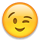#### Howdy, Stranger!

It looks like you're new here. Sign in or register to get started.

# Get around divide by zero.

edited 2005-01-13
Here is an expression that returns 1 when x is zero (or the original value if it is not zero)

x = figure below division line, also figure below division has max 2 decimals
The 100 below is to cater for -0.01 <= x <= 0.01
```new value := x + 1 - round(x*100 / (x*100+1))
```

Regards,
gus

• But wont it do a div with zero if x=-0.01And if x=1 or -1 it returns 1.01 or -1.01

I don't understand what you are trying to do :-( Just to make sure - are you trying to make this logic, but as a calcultaion?
if x=0 then x=1;
Regards
Peter
• Correct, if x=0 then x=1 without the use of an if statement.
• If you found the solution I think it is something which I cannot recommand for use. The understandability of the code will be too low... If is faster and clear...
And in many cases if you divide with 0 it means that there is some problem around...
Kamil Sacek
MVP - Dynamics NAV
My BLOG
NAVERTICA a.s.